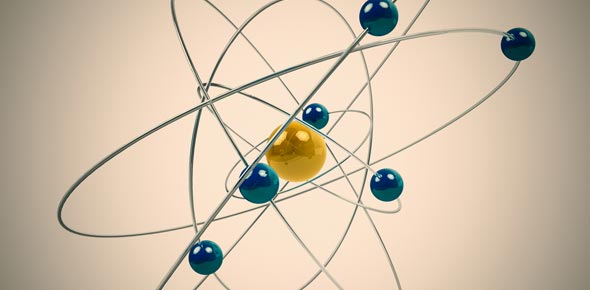# +2 Physics Unit 8 - Nuclear Physics

19 QuestionsSettingsPrepared by P. ILAIYARAJA M. Sc. ,B. Ed. , PG ASST IN PHYSICS , GHSS, PANCHANATHIKKULAM EAST, NAGAI DT.   ; &www. Padasalai. Net

Questions and Answers
• 1.
The nuclear radius of nucleus is
• A.

(2)

• B.

(1)

• C.

(3)

• D.

(4)

• 2.
The nuclei are example of
• A.

Isotones

• B.

Isomers

• C.

Isotopes

• D.

isobars

• 3.
The mass defect of a certain nucleus is found to be 0.03 amu. Its binding energy is
• A.

27.93 MeV

• B.

27.93 eV

• C.

27.93 GeV

• D.

27.93 KeV

• 4.
Nuclear fission can be explained by
• A.

Liquid drop model

• B.

Quark model

• C.

Shell model

• D.

Bohr atom model

• 5.
The nucleons in a nucleus are attracted by
• A.

Nuclear force

• B.

Magnetic force

• C.

Gravitational force

• D.

Electrostatic force

• 6.
The ionisation power is maximum for
• A.

α – particles

• B.

γ – rays

• C.

β − particles

• D.

Neutrons

• 7.
The half life period of a certain radioactive element with disintegration constant 0.0693 per day is
• A.

10 days

• B.

14 days

• C.

140 days

• D.

1.4 days

• 8.
The radio-isotope used in agriculture is
• A.

(2)

• B.

(3)

• C.

(4)

• D.

(1)

• 9.
The average energy released per fission is
• A.

200 MeV

• B.

200 meV

• C.

200 GeV

• D.

200 eV

• 10.
The explosion of atom bomb is based on the principle of
• A.

uncontrolled fission reaction

• B.

Controlled fission reaction

• C.

Fusion reaction

• D.

Thermonuclear reaction

• 11.
Anaemia can be diagnosed by
• A.

(3)

• B.

(2)

• C.

(1)

• D.

(4)

• 12.
In the nuclear reaction ,  X-stands for
• A.

Neutron

• B.

Proton

• C.

Electron

• D.

Deutron

• 13.
In β – decay
• A.

Neutron number decreases by one

• B.

Atomic number decreases by one

• C.

mass number decreases by one

• D.

Proton number remains the same

• 14.
Isotopes have
• A.

Same proton number but different neutron number

• B.

Same proton number and neutron number

• C.

Same mass number but different atomic number

• D.

Same neutron number but different proton number

• 15.
The time taken by the radioactive element to reduce to 1/e times is
• A.

Mean life

• B.

Half life/2

• C.

Half life

• D.

Twice the mean life

• 16.
The half life period of is 10.1 minute. Its life time is
• A.

(4)

• B.

(3)

• C.

(2)

• D.

(1)

• 17.
Positive rays of the same element produce two different traces in a Bainbridge mass spectrometer. The positive ions have
• A.

Different mass with same velocity

• B.

Different mass with different velocity

• C.

Same mass with same velocity

• D.

Same mass with different velocity

• 18.
The binding energy of  nucleus is
• A.

493 MeV

• B.

8.8 MeV

• C.

88 MeV

• D.

41.3 MeV

• 19.
The ratio of nuclear density to the density of mercury is about
• A.

(3)

• B.

(2)

• C.

(4)

• D.

(1)

More Nuclear Physics Quizzes
Related Topics